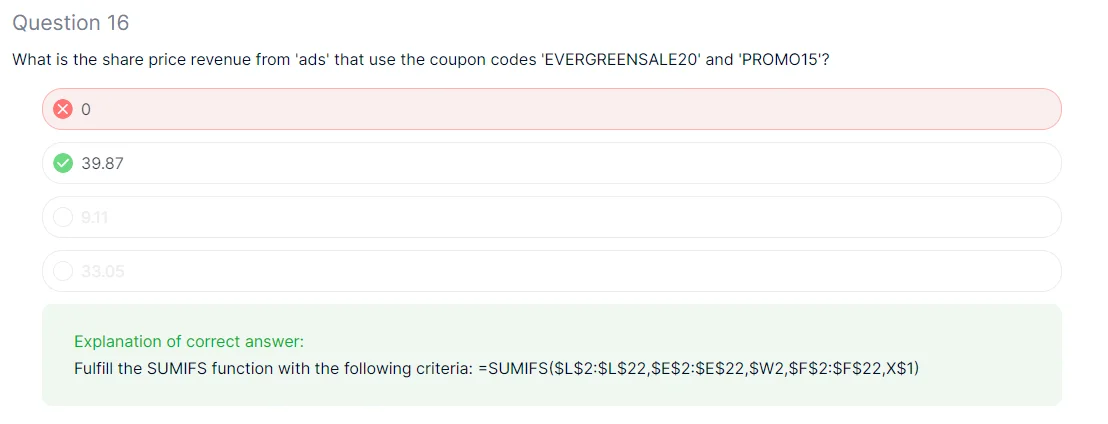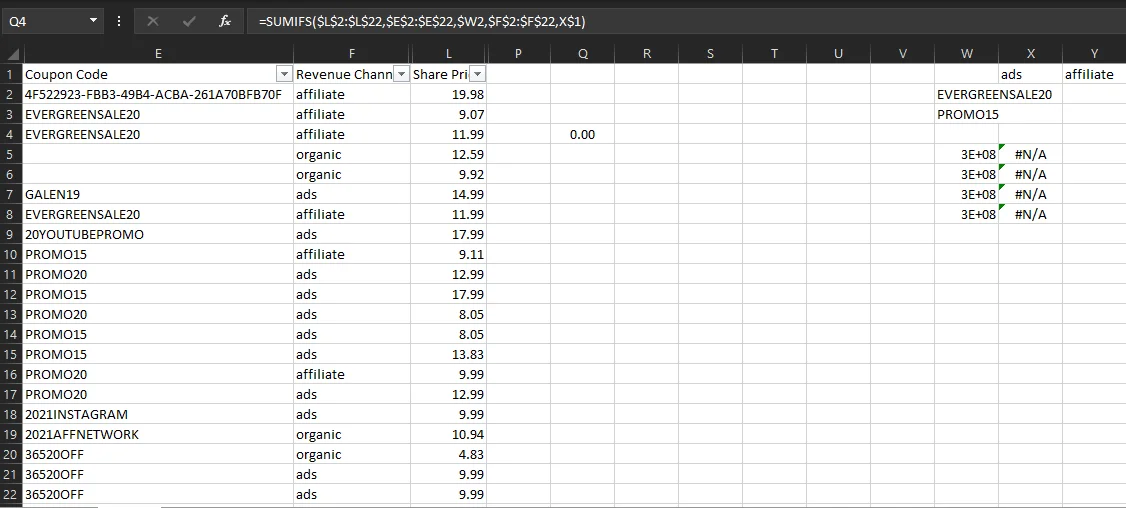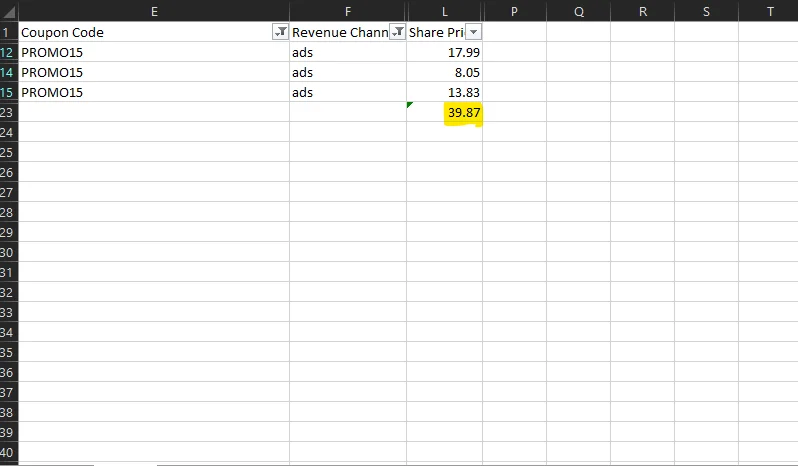01 Oct 2022

Posted on:

30 Sept 2022

1

# Resolved:Where is the Wrong here?

Could you please advise where is the wrong in the written function as it the same as the correct solution but when i use it, it return with Zero not 39.37?Instructor
Posted on:

30 Sept 2022

0

Hello,
The screenshot, which you've shared doesn't allow me to see column E. Probably Excel didn't recognize one of your criteria and this is why the result of the function is 0. I need to see columns E and W to be able to tell you the mistake.
Best,
Ned

Posted on:

30 Sept 2022

0

This is the full picture of the columns related to the functionIf we manually filtered the requested criteria it shown that no ads revenue related to "EVERGREENSALE20" code
and the result 39.87 related only to the total revenue of "PROMO15 code as per the belowInstructor
Posted on:

30 Sept 2022

0

Oh right. And the sum of the two is 0 + 39.87 = 39.87. Correct?

Posted on:

30 Sept 2022

0

Yes, Correct but how we can apply this with one formula to get the correct result of 39.87?

Instructor
Posted on:

01 Oct 2022

0

You can obtain the answer for both rows and then sum the two answers. Does that work?

Posted on:

01 Oct 2022

0

let me clarify my understanding:

we can't use the same criteria range twice for Sumifs function, so we use Sumifs for two times first one for EVERGREENSALE20 and the second for PROMO15 then we sum the two answers

Am i correct?

Instructor
Posted on:

01 Oct 2022

1

You can actually use just SUMIF in this case I think.
Best,
Ned

``````
``````